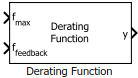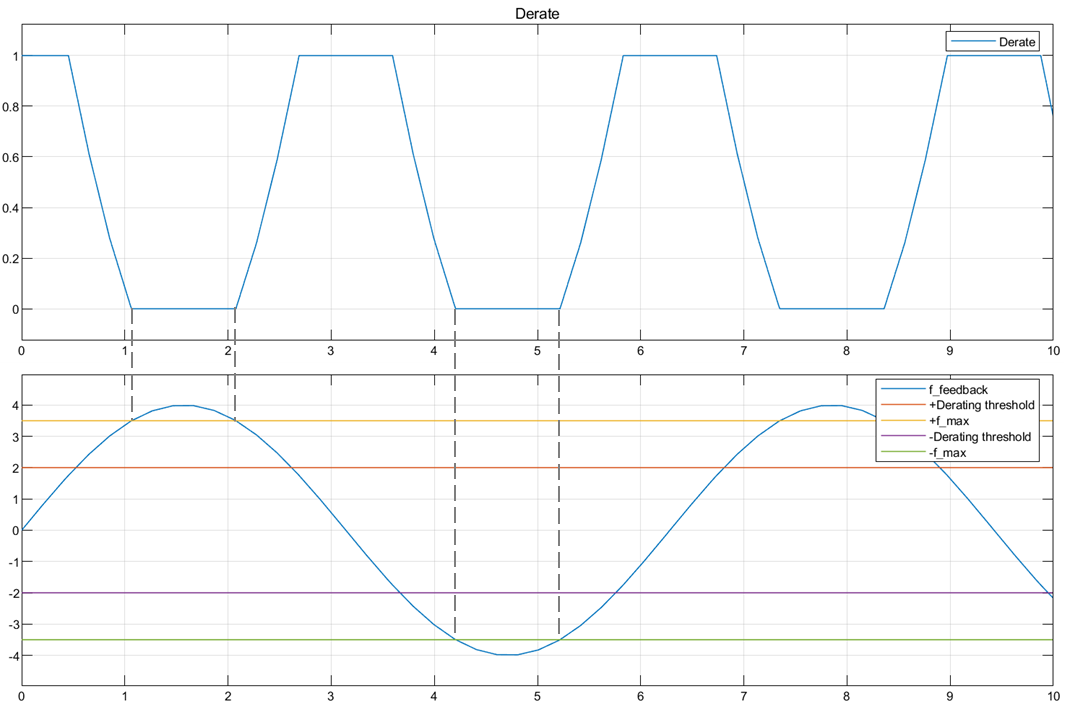# Derating Function

Compute derating factor

• Library:
• Motor Control Blockset / Controls / Controllers

•## Description

The Derating Function block generates the derating factor (`y`) according to the feedback (`ffeedback`) and maximum limit (`fmax`) values of the input reference signal.

The derating factor:

• Remains equal to one when `ffeedback` lies between positive and negative values of the Derating threshold. The derating factor varies linearly outside this range according to `ffeedback`.

• Remains equal to zero when the reference signal lies beyond (positive or negative) `fmax`.

Therefore, you can use the generated derating factor to derate a control signal after the reference signal crosses the specified Derating threshold.

This figure shows the block output when you use a sinusoidal wave as `ffeedback`.### Equations

The Derating threshold parameter, $x$ indicates the percentage of peak amplitude for the reference signal. The Derating threshold is 0.5 in the block output shown, which results in a threshold value of 2 (for the peak amplitude value of 4 for the sinusoidal reference signal).

`$x=\left[0,1\right)$`

This equation describes how the block computes the derating factor (`y`).

## Ports

### Input

expand all

Maximum limit of the reference signal value beyond which the derating factor becomes zero.

Data Types: `single` | `double` | `fixed point`

Reference signal that the block uses to generate the derating factor, which you can then use to derate a control signal.

Data Types: `single` | `double` | `fixed point`

### Output

expand all

Derating factor that the block generates based on the feedback and maximum limit values of the reference signal when the signal exceeds the value of the Derating threshold parameter.

Data Types: `single` | `double` | `fixed point`

## Parameters

expand all

The reference signal value beyond which the block generates the derating factor.

Get ebook